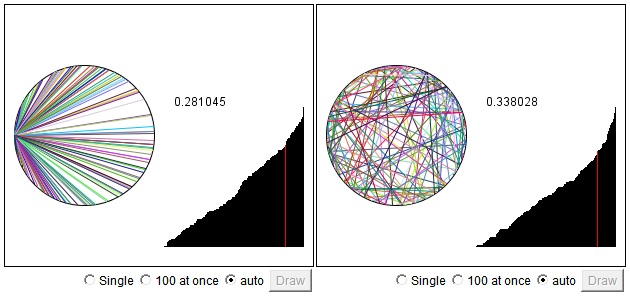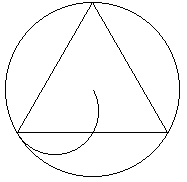Theory of Probability (much as the rest of Mathematics) is actually a recent invention. And the development has not been smooth at all. The first attempts to formalize the calculus of probability were due to marquis de Laplace (1749-1827) who proposed to define the probability p(A) of an outcome A as the ratio of the number of events that result in the outcome A to the total number of possible events. This is of course only meaningful if the number of all possible events is finite and, in addition, all the events are equiprobable. The notion which Laplace has also defined. All elementary Probability courses depend on that definition. However note that the definition is, in a sense, circular - a notion of equiprobable is defined prior to the introduction of probable.

Thus, at the time, the field did not seem to have a sound foundation. Attempts to extend the definition to the case of infinite number of events led to even greater difficulties. The Bertrand's Paradox is one such discovery that made mathematicians wary of the whole notion of probability.

Consider the following problem:

Given a circle. Find the probability that a chord chosen at random be longer than the side of an inscribed equilateral triangle.

The problem has apparently several solutions.

### First Solution.

We have to choose randomly two points on a circle and measure the distance between the two. Therefore, the only important thing is the position of the second point relative to the first one. In other words, position of the first point has no effect on the outcome. Thus let us fix the point A and consider only the chords that emanate from this point. Then it becomes clear that 1/3 of the outcomes will result in a chord longer than the side of an equilateral triangle.### Second Solution

A chord is fully determined by its midpoint. Chords whose length exceeds the side of an equilateral triangle have their midpoints inside a smaller circle with radius equal to 1/2 that of the given one. Hence, its area is 1/4 of the big circle which also defines the proportion of favorable outcomes - 1/4.### Third Solution

A chord is fully determined by its midpoint. Chords whose length exceeds the side of an equilateral triangle have their midpoints closer to the center than half the radius. If the midpoints are distributed uniformly over the radius (instead of over the area, as was the case in the second solution), the probability becomes 1/2.Below there are two simulations that illustrate the approach adopted in the first solution. In one, two points are randomly chosen on the circle and then connected by a chord. In another, one point is fixed and another is selected randomly. Assuming the radius of the circle is 1, the side of the triangle is3 while the biggest chord (diameter) has length of 2. The diagram to the right of circles represents empirical probability distribution of the chord length. The graph is defined for values between 0 and 2. The value of the graph at a point x is an estimated probability of a chord to be not longer than x. The maximum value of 1 is attained at the right end since no chord may be longer than 2. The red line indicates the position of3.If you are reading this, your browser is not set to run Java applets. Try IE11 or Safari and declare the site https://www.cut-the-knot.org as trusted in the Java setup.What if applet does not run?### An aside

The two simulations above performed by the same applet. By passing different values to a parameter ShouldFix (see the source for this HTML file) the applet executes different simulations. Being a multi-tasking environment, Java lets two copies of the same applet run simultaneously.The applet demonstrates (but does not prove) that the reasoning applied in the first solution is very sound. Probability distributions in both cases appear to be equal. So that the first solution seems quite plausible. This is where we'll start.

 Assume the big circle has radius R and let A denote the left vertex of the triangle. Midpoints of the chords with one end at A lie on a circle of radius R/2 tangent to the big one at the point A. This small circle is split by the triangle into three equal parts. Thus, if we look into the distribution of midpoints of the chords in the first solution, we'll find that favorable outcomes occur in 1/3 of all cases - in complete agreement with the derivation.Now let us consider one half of the small circle. By the base of the triangle it's divided in the ratio 1:2. One part contains midpoints of chords longer than the side of the triangle whereas two parts contain midpoints of chords that are shorter than the side of the triangle.Let us rotate this arc around the center of the big circle. It's possible to sweep the whole of the circle without covering any point (accept for the center) more than once. The 'favorable' part of the arc will sweep a circle of radius R/2 with the same center - it's the one that appeared in the second solution. Perhaps naively one would expect that 1/3 of the arc will sweep twice as small an area as swept by the remaining 2/3 of the arc. As we saw, the proportion is actually 1:3 and not 1:2! It appears that the midpoints of chords in a circle whose endpoints are uniformly distributed on the circumference are more densely located near its center than towards its periphery. Thus the "paradox" merely indicates that probability distributions in two dimensions warrant a more careful consideration than the second solution suggested. The notion of uniform randomness is actually less obvious than appeared at the first glance.Below are the simulations of the second and third solutions. In both cases, I randomly choose a point inside the circle and display the chord perpendicular to the radius-vector to that point. The difference between the two simulations is in that, for the third solution, the point is defined in its polar coordinates (radius+angle) which are selected independently, while, for the second solution, the point is defined by its Cartesian coordinates. In the latter case, x and y are chosen randomly and independently in the square circumscribed around the circle. Points that fall outside the circle are being discarded.

 If you are reading this, your browser is not set to run Java applets. Try IE11 or Safari and declare the site https://www.cut-the-knot.org as trusted in the Java setup.What if applet does not run?## Reference

• M. Gardner, The Colossal Book of Mathematics, W. W. Norton & Co, 2001, Ch. 21: Probability and Ambiguity
• M.Kac and S.M.Ulam, Mathematics and Logic, Dover Publications, NY, 1968.
• F. Mosteller, Fifty Challenging Problems in Probability with Solutions , Dover, 1987, problem 25### Geometric Probability